# Plotting of Data using Generic plots in R Programming – plot() Function

• Last Updated : 07 Dec, 2021

plot() function in R Programming Language is defined as a generic function for plotting. It can be used to create basic graphs of a different type.

Syntax: plot(x, y, type)

Parameters

• x and y: coordinates of points to plot
• type: the type of graph to create

Returns: different type of plots

## R

 `plot``(3, 4)`

Output: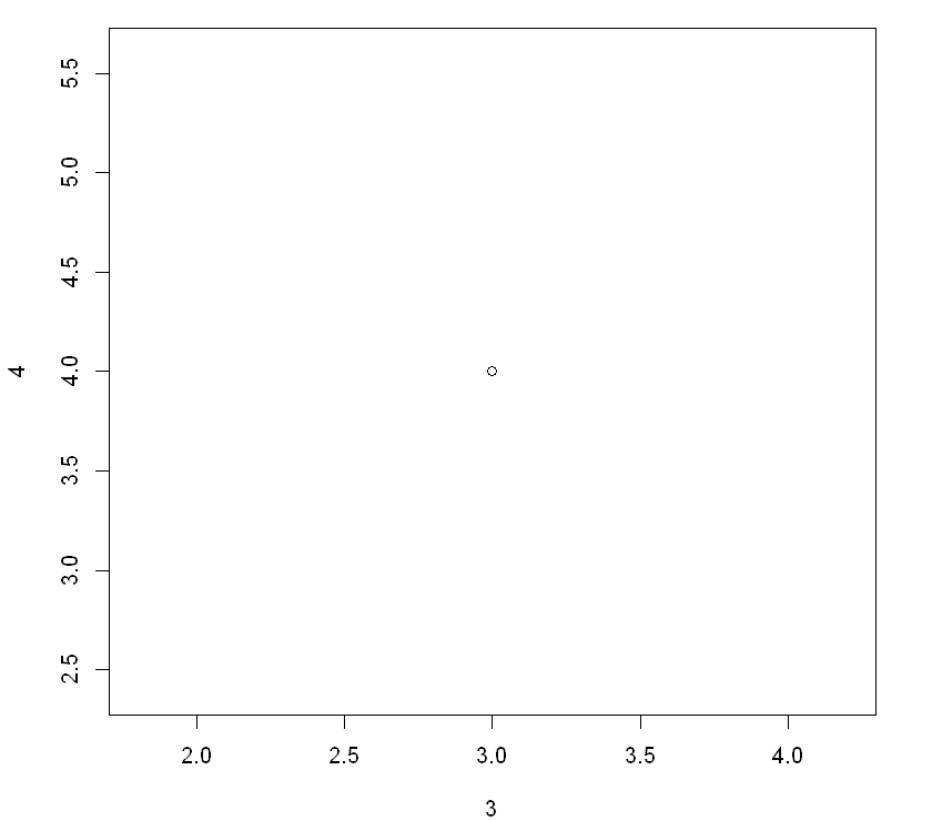## R

 `plot``(``c``(1, 3, 4), ``c``(4, 5 , 8))`

Output: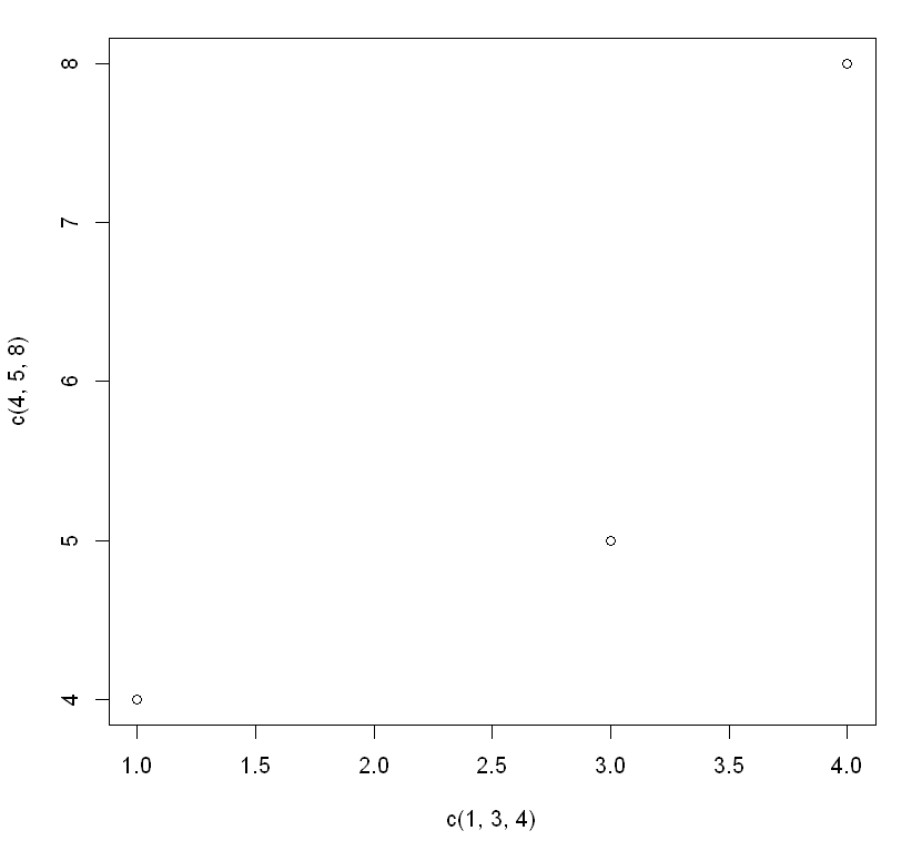## R

 `plot``(1:20)`

Output: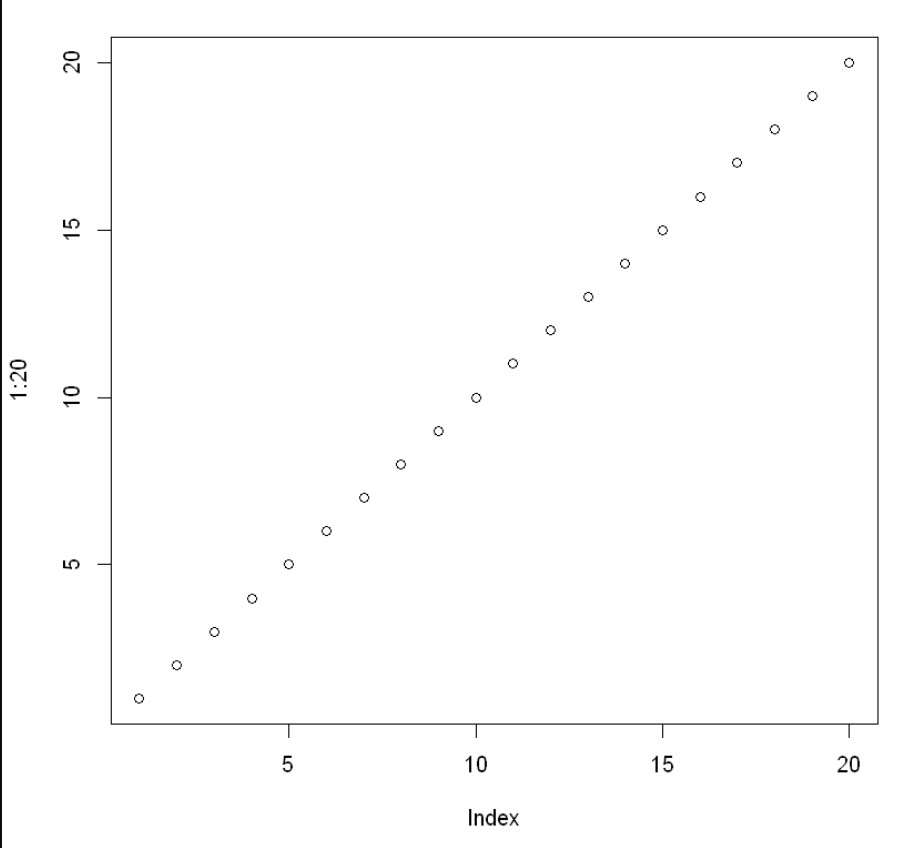## R

 `# Values for x and y axis``x <- 1:5; y = x * x` `# Using plot() function``plot``(x, y, type = "l")``plot``(x, y, type = "h")`

Output: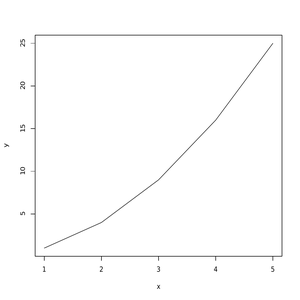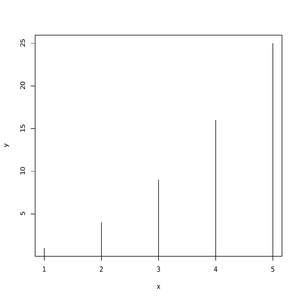In the above example type=“l” stands for lines graph, type=“h” stands for ‘histogram’ like vertical lines.

## R

 `# R program to plot a graph` `# Creating x and y-values``x - 1:5; y = x * x` `# Using plot function``plot``(x, y, type = "b")``plot``(x, y, type = "s")``plot``(x, y, type = "p")`

Output: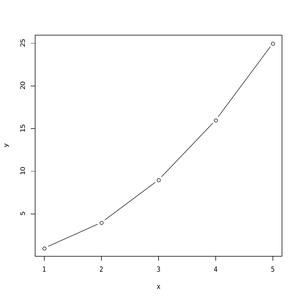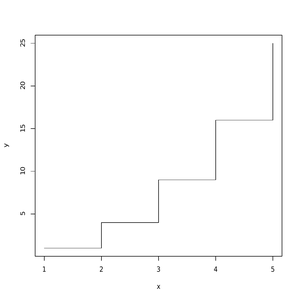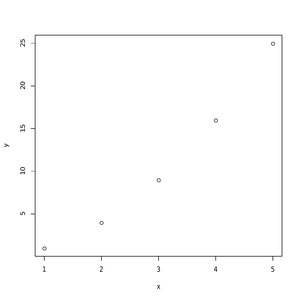Here, in the above example type=“b” stands for both that means points are connected by a line

type=“s”indicates stair steps

type=“p” for points (by default).

My Personal Notes arrow_drop_up